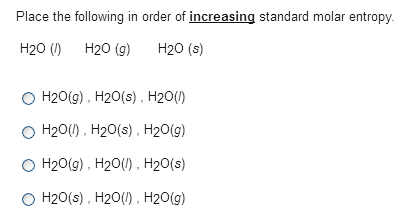# Problem: Place the following in order of increasing standard molar entropy.H2O(l) H2O(g) H2O(s) a. H2O(g), H2O(s), H2O(l) b. H2O(l), H2O(s), H2O(g) c. H2O(g), H2O(l), H2O(s) d. H2O(s), H2O(l), H2O(g)

###### FREE Expert Solution
98% (453 ratings)###### Problem Details

Place the following in order of increasing standard molar entropy.

H2O(l) H2O(g) H2O(s)

a. H2O(g), H2O(s), H2O(l)

b. H2O(l), H2O(s), H2O(g)

c. H2O(g), H2O(l), H2O(s)

d. H2O(s), H2O(l), H2O(g)